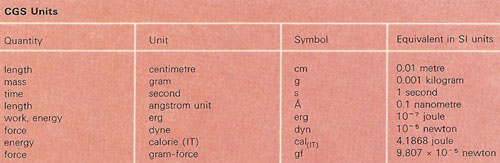A# CGS unitsCGS units are a system of units derived, like MKS units, from the metric system, and based on the centimeter (length), gram (mass), and second (time). The CGS system was introduced formally by the British Association for the Advancement of Science in 1874. It has now been replaced for most scientific purposes by the SI system. However, it is still common to see some CGS units used, including the dyne (force), erg (energy), and gauss (magnetic field density).

Several variants of CGS units are used for electrical and magnetic problems, including electrostatic units (esu or stat-units), electromagnetic units (emu or ab-units) and the Gaussian system. In this last, ab-units are for quantities arising primarily in an electromagnetic context, stat-units for electrostatic quantities and both the permeability and the permittivity of free space are set equal to unity. As a result, the electromagnetic constant (c) tends to occur in equations in which electrostatic and magnetic quantities are mixed.